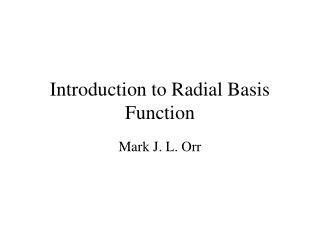DownloadDownload PresentationIntroduction to Radial Basis Function

# Introduction to Radial Basis Function

Télécharger la présentation## Introduction to Radial Basis Function

- - - - - - - - - - - - - - - - - - - - - - - - - - - E N D - - - - - - - - - - - - - - - - - - - - - - - - - - -
##### Presentation Transcript

1. Introduction to Radial Basis Function Mark J. L. Orr

2. Radial Basis Function Networks • Linear model

3. Radial functions • Gassian RBF:c : center, r : radius • monotonically decreases with distance from center • Multiquadric RBF • monotonically increases with distance from center

4. Gaussian RBF multiqradric RBF

5. Least Squares • model • training data : {(x1, y1), (x2, y2), …, (xp, yp)} • minimize the sum-squared-error

6. Example • Sample points (noisy) from the curve y = x : {(1, 1.1), (2, 1.8), (3, 3.1)} • linear model : f(x) = w1h1(x) + w2h2(x),where h1(x) = 1, h2(x) = x • estimate the coefficient w1, w2

7. f(x) = x

8. New model : f(x) = w1h1(x) + w2h2(x) + w3h3(x)where h1(x) = 1, h2(x) = x, h3(x) = x2

9. absorb all the noise : overfit • If the model is too flexible, it will fit the noise • If it is too inflexible, it will miss the target

10. The optimal weight vector • model • sum-squared-error • cost function : weight penalty term is added

11. Example • Sample points (noisy) from the curve y = x : {(1, 1.1), (2, 1.8), (3, 3.1)} • linear model : f(x) = w1h1(x) + w2h2(x),where h1(x) = 1, h2(x) = x • estimate the coefficient w1, w2

12. The projection matrix • At the optimal weight:the value of cost function C = yTPythe sum-squared-error S = yTP2y

13. Model selection criteria • estimates of how well the trained model will perform on future input • standard tool : cross validation • error variance

14. Cross validation • leave-one-out (LOO) cross-validation • generalized cross-validation

15. Ridge regression • mean-squared-error

16. Global ridge regression • Use GCV • re-estimation formula • initialize  • re-estimate , until convergence

17. Local ridge regression • research problem

18. Example

19. Selection the RBF • forward selection • starts with an empty subset • added one basis function at a time • most reduces the sum-squared-error • until some chosen criterion stops • backward elimination • starts with the full subset • removed one basis function at a time • least increases the sum-squared-error • until the chosen criterion stops decreasing